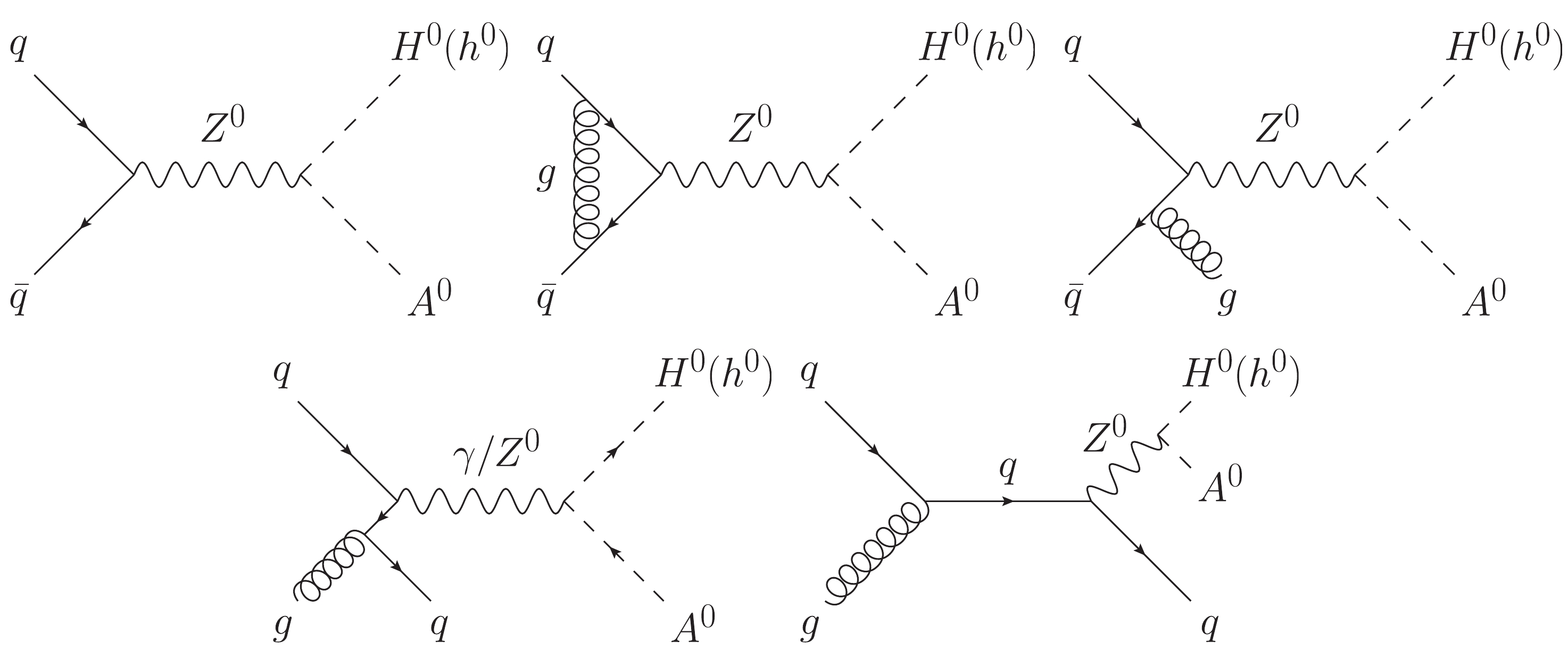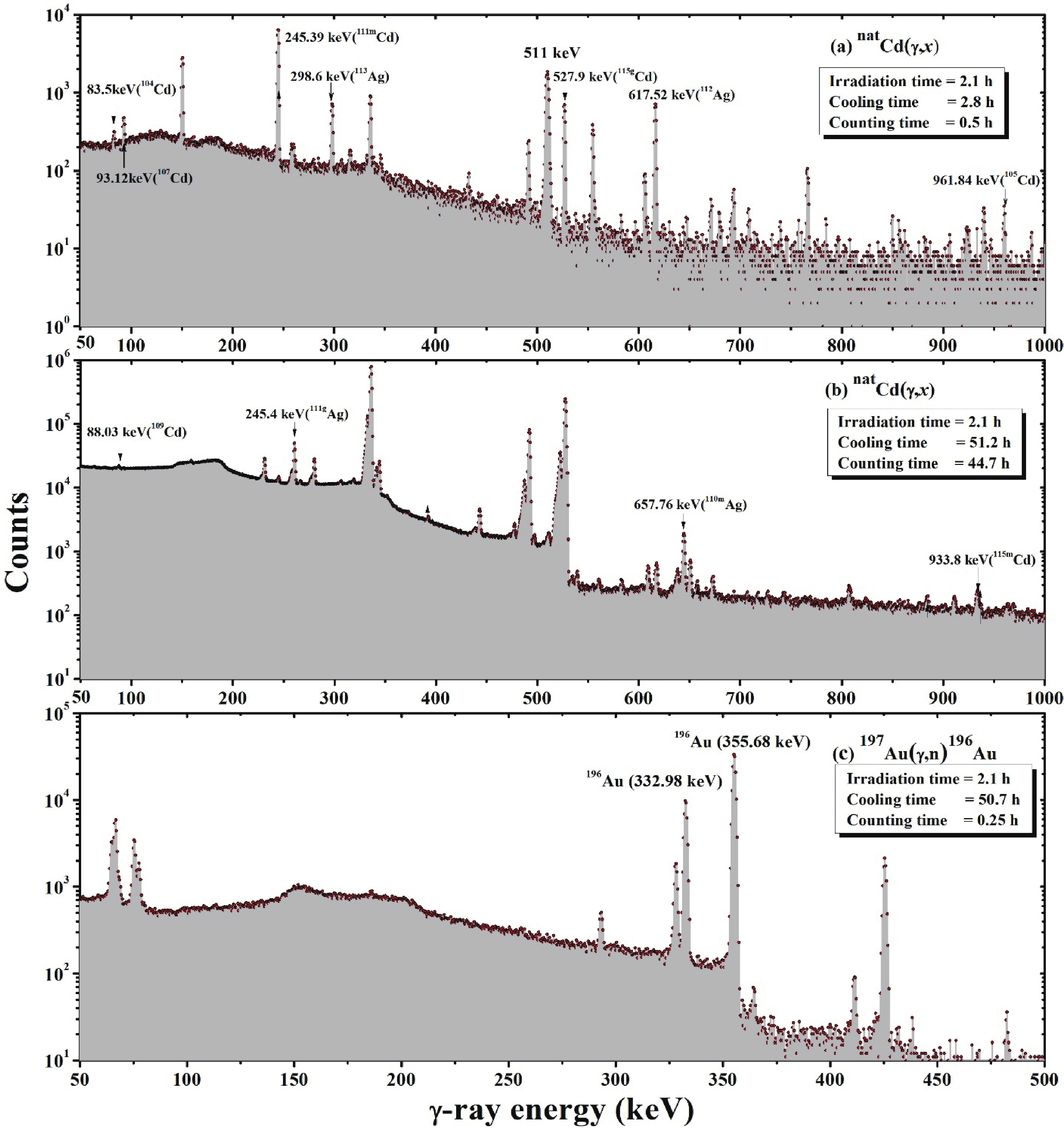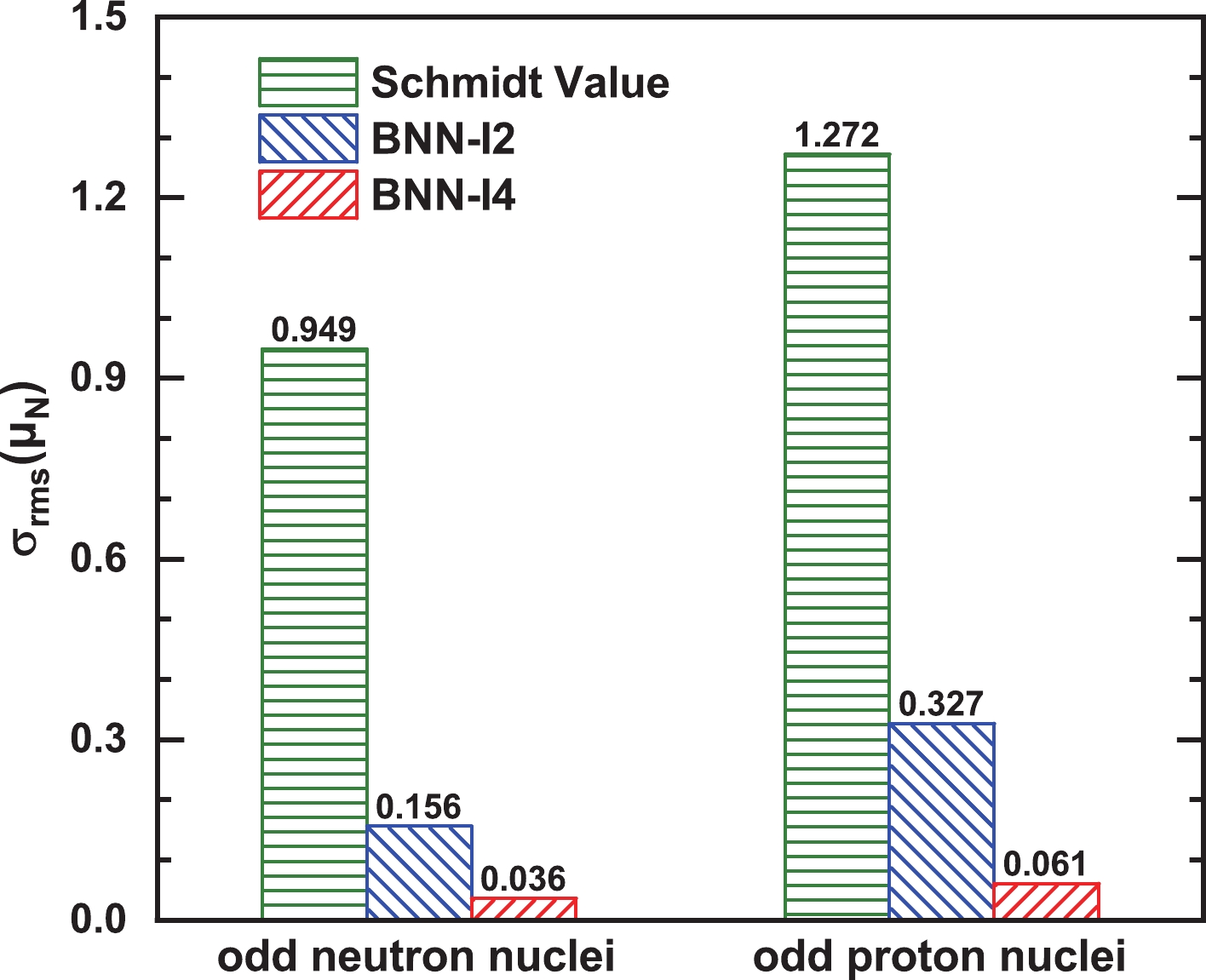Highlights
• Scalar-pseudoscalar pair production at the Large Hadron Collider at NLO+NLL accuracy in QCD2021, 45(12): 123102. doi: 10.1088/1674-1137/ac23d2We thoroughly investigate both transverse momentum and threshold resummation effects on scalar-pseudoscalar pair production via quark-antiquark annihilation at the $13 \; \text{TeV}$Large Hadron Collider at QCD NLO+NLL accuracy. A factorization method is introduced to properly supplement the soft-gluon (threshold) resummation contribution from parton distribution functions to the resummed results obtained by the Collins-Soper-Sterman resummation approach. We find that the impact of the threshold-resummation improved PDFs is comparable to the resummation effect of the partonic matrix element and can even predominate in high invariant mass regions. Moreover, the loop-induced gluon-gluon fusion channel in the type-I two-Higgs-doublet model is considered in our calculations. The numerical results show that the electroweak production via quark-antiquark annihilation dominates over the gluon-initiated QCD production by $1 \sim 2$orders of magnitude.
• Measurements of natCd(γ, x) reaction cross sections and isomer ratio of 115m,gCd with the bremsstrahlung end-point energies of 50 and 60 MeV2021, 45(12): 124002. doi: 10.1088/1674-1137/ac256bThe flux-weighted average cross sections of natCd(γ, xn)115g,m,111m,109,107,105,104Cd and natCd(γ, x)113g,112,111g,110mAg reactions were measured at the bremsstrahlung end-point energies of 50 and 60 MeV. The activation and off-line γ-ray spectrometric technique was carried out using the 100 MeV electron linear accelerator at the Pohang Accelerator Laboratory, Korea. The natCd(γ, xn) reaction cross sections as a function of photon energy were theoretically calculated using the TALYS-1.95 and the EMPIRE-3.2 Malta codes. Then, the flux-weighted average cross sections were obtained from the theoretical values of mono-energetic photons. These values were compared with the flux-weighted values from the present study and were found to be in general agreement. The measured experimental reaction cross-sections and integral yields were described for cadmium and silver isotopes in the natCd(γ, xn)115g,m,111m,109,107,105,104Cd and natCd(γ, x)113g,112,111g,110mAg reactions. The isomeric yield ratio (IR) of 115g,mCd in the natCd(γ, xn) reaction was determined for the two bremsstrahlung end-point energies. The measured isomeric yield ratios of 115g,mCd in the natCd(γ, xn) reaction were also compared with the theoretical values of the nuclear model codes and previously published literature data of the 116Cd(γ, n) and 116Cd(n, 2n) reactions. It was found that the IR value increases with increasing projectile energy, which demonstrates the characteristic of excitation energy. However, the higher IR value of 115g,mCd in the 116Cd(n, 2n) reaction compared to that in the 116Cd(γ, n) reaction indicates the role of compound nuclear spin alongside excitation energy.
• Magnetic moment predictions of odd-A nuclei with the Bayesian neural network approach2021, 45(12): 124107. doi: 10.1088/1674-1137/ac28f9The Bayesian neural network approach has been employed to improve the nuclear magnetic moment predictions of odd-A nuclei. The Schmidt magnetic moment obtained from the extreme single-particle shell model makes large root-mean-square (rms) deviations from data, i.e., 0.949 $\mu_\mathrm{N}$and 1.272 $\mu_\mathrm{N}$for odd-neutron nuclei and odd-proton nuclei, respectively. By including the dependence of the nuclear spin and Schmidt magnetic moment, the machine-learning approach precisely describes the magnetic moments of odd-A nuclei with rms deviations of 0.036 $\mu_\mathrm{N}$for odd-neutron nuclei and 0.061 $\mu_\mathrm{N}$for odd-proton nuclei. Furthermore, the evolution of magnetic moments along isotopic chains, including the staggering and sudden jump trend, which are difficult to describe using nuclear models, have been well reproduced by the Bayesian neural network (BNN) approach. The magnetic moments of doubly closed-shell $\pm1$nuclei, for example, isoscalar and isovector magnetic moments, have been well studied and compared with the corresponding non-relativistic and relativistic calculations.
In Press
More >
• Toward discovering low-lying P-wave excited Σc baryon statesPublished: 2021-12-03, doi: 10.1088/1674-1137/ac3123
Show Abstract
In this study, by combining the equal spacing rule with recent observations of $\Omega_c(X)$and $\Xi_c(X)$baryons, we predict the spectrum of the low-lying $\lambda$-mode $1P$-wave excited $\Sigma_c$states. Furthermore, their strong decay properties are predicted using the chiral quark model and the nature of $\Sigma_c(2800)$is investigated by analyzing the $\Lambda_c\pi$invariant mass spectrum. The $\Sigma_c(2800)$structure observed in the $\Lambda_c \pi$mass spectrum was found to potentially arise from two overlapping $P$-wave $\Sigma_c$resonances, $\Sigma_c(2813)3/2^-$and $\Sigma_c(2840)5/2^-$. These resonances have similar decay widths of $\Gamma\sim 40$MeV and predominantly decay into the $\Lambda_c \pi$channel. The $\Sigma_c(2755)1/2^-$state is likely to be a very narrow state with a width of $\Gamma\sim 15$MeV, with its decays almost saturated by the $\Lambda_c \pi$channel. Additionally, evidence of the $\Sigma_c(2755) {1}/{2}^-$resonance as a very narrow peak may be seen in the $\Lambda_c\pi$invariant mass spectrum. The other two $P$-wave states, $\Sigma_c(2746) {1}/{2}^-$and $\Sigma_c(2796) {3}/{2}^-$, are relatively narrow states with similar widths of $\Gamma\sim 30$MeV and predominantly decay into $\Sigma_c\pi$and $\Sigma^{*}_c\pi$, respectively. This study can provide useful references for discovering these low-lying $P$-wave states in forthcoming experiments.
• Phase structures of neutral dense quark matter and applicationto strange starsPublished: 2021-11-25, doi: 10.1088/1674-1137/ac2f95
Show Abstract
In the contact interaction model, the quark propagator has only one solution, namely, the chiral symmetry breaking solution, at vanishing temperature and density in the case of physical quark mass. We generalize the condensate feedback onto the coupling strength from the 2 flavor case to the 2+1 flavor case, and find the Wigner solution appears in some regions, which enables us to tackle chiral phase transition as two-phase coexistences. At finite chemical potential, we analyze the chiral phase transition in the conditions of electric charge neutrality and $\beta$equilibrium. The four chemical potentials, $\mu_u$, $\mu_d$, $\mu_s$and $\mu_e$, are constrained by three conditions, so that one independent variable remains: we choose the average quark chemical potential as the free variable. All quark masses and number densities suffer discontinuities at the phase transition point. The strange quarks appear after the phase transition since the system needs more energy to produce a $d$-quark than an $s$-quark. Taking the EOS as an input, the TOV equations are solved numerically, and we show that the mass–radius relation is sensitive to the EOS. The maximum mass of strange quark stars is not susceptible to the parameter $\Lambda_q$we introduced.
• Model-independent Veltman condition, naturalness and the little hierarchy problemPublished: 2021-11-24, doi: 10.1088/1674-1137/ac2ffa
Show Abstract
We adopt a bottom-up Effective Field Theory (EFT) approach to derive a model-independent Veltman condition to cancel out the quadratic divergences in the Higgs mass. We show using the equivalence theorem that all the deviations in the Higgs couplings to the $W$and $Z$from the SM predictions should vanish. We argue based on tree-level unitarity that any new physics that naturally cancels out the quadratic divergences should be $\lesssim 19$TeV. We show that the level of fine-tuning required is $O(0.1\%-1\%)$unless the UV sector has a symmetry that forces the satisfaction of the model-independent Veltman condition, in which case all fine-tuning is eliminated. We also conjecture that, if no new physics that couples to the Higgs is observed up to $\sim 19$TeV, or if the Higgs couplings to the SM particles conform to the SM predictions, then the Higgs either does not couple to any UV sector or is fine-tuned.
Archive